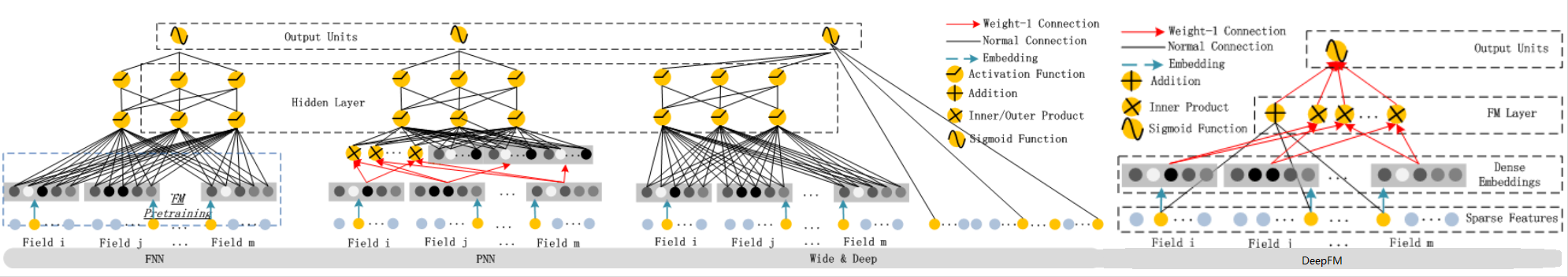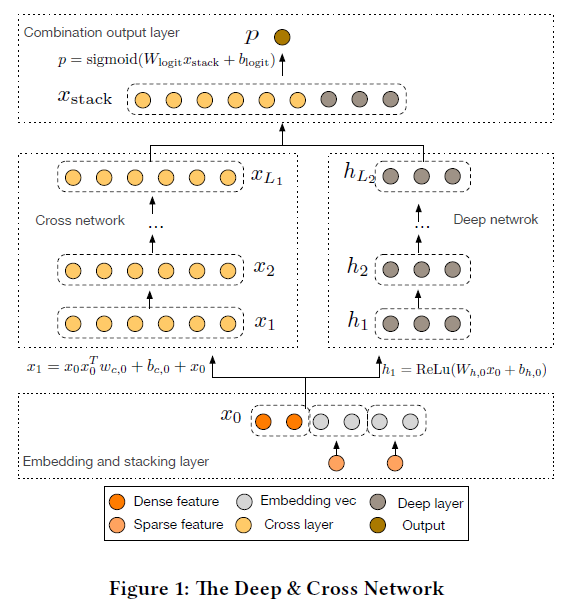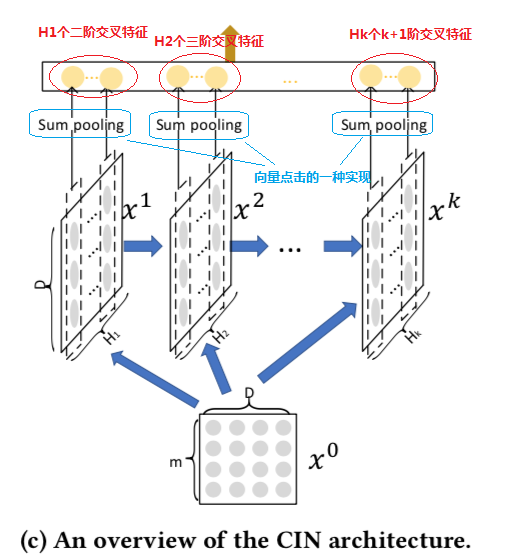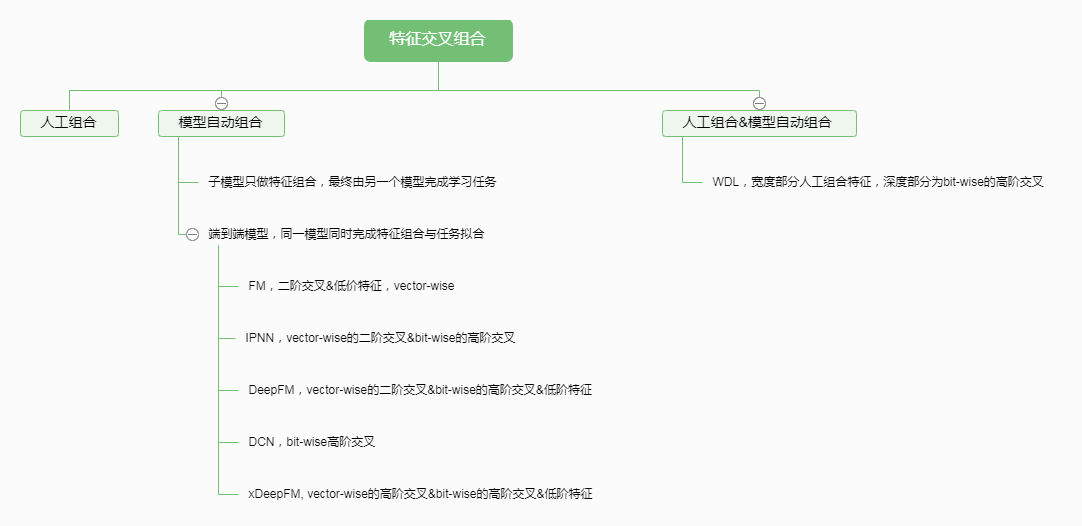1. 第一条主脉络是以FM家族为代表的深度模型，它们的共同特点是自动学习从原始特征交叉组合新的高阶特征。
2. 第二条主脉络是一类使用attention机制处理时序特征的深度模型，以DIN、DIEN等模型为代表。
3. 第三条主脉络是以迁移学习、多任务学习为基础的联合训练模型或pre-train机制，以ESMM、DUPN等模型为代表。

特征组合的演化路线

FM模型说起，FM通过特征对之间的隐变量内积来提取特征组合，其函数形式如下：

MLP（plain-DNN）因其特殊的结构天然就具有学习高阶特征组合的能力，它可以在一定的条件下以任意精度逼近任意函数。然而，plain-DNN以一种隐式的方式建模特征之间的交互关系，我们无法确定它学习到了多少阶的交叉关系。高维稀疏的原始特征在输入给DNN之前一般都会经过embedding处理，每一个域（类别）的原始特征都会被映射到一个低维稠密的实数向量，称之为embedding向量。FM模型中的隐向量也可以理解为embedding向量。Embedding向量中的元素用术语bit表示，可以看出plain-DNN的高阶特征交互建模是元素级的（bit-wise），也就是说同一个域对应的embedding向量中的元素也会相互影响。这与FM显式构建特征交叉关系的方式是不一样的，FM类方法是以向量级（vector-wise）的方式来构建高阶交叉关系。经验上，vector-wise的方式构建的特征交叉关系比bit-wise的方式更容易学习。FM、DeepFM和Inner-PNN都是通过原始特征隐向量的内积来构建vector-wise的二阶交叉特征，这种方式有两个主要的缺点：

1. 必须要穷举出所有的特征对，即任意两个field之间都会形成特征组合关系，而过多的组合关系可能会引入无效的交叉特征，给模型引入过多的噪音，从而导致性能下降。
2. 二阶交叉特征有时候是不够的，好的特征可能需要更高阶的组合。虽然DNN部分可以部分弥补这个不足，但bit-wise的交叉关系是晦涩难懂、不确定并且不容易学习的。

DCN模型以一个嵌入和堆叠层(embedding and stacking layer)开始，接着并列连一个cross network和一个deep network，接着通过一个combination layer将两个network的输出进行组合。交叉网络（cross network）的核心思想是以有效的方式应用显式特征交叉。交叉网络由交叉层组成，每个层具有以下公式：

• $x_l,x_{l+1}$是列向量（column vectors），分别表示来自第$l$层和第($l+1$)层cross layers的输出；
• $w_l, b_l \in R^d$是第$l$层layer的weight和bias参数。DCN模型中使用的这种“压缩”机制是完美的吗，有没有什么局限性？实际上这种“压缩”方式把特征交互关系限定在一种特殊的形式上。我们再来看看cross layer的计算公式，为了简化，以便说明问题，下面去掉偏置项。

1. CrossNet的输出被限定在一种特殊的形式上
2. 特征交叉还是以bit-wise的方式构建的

极深因子分解机模型（xDeepFM）

xDeepFM模型是自动构建交叉特征且能够端到端学习的集大成者，它很好的回答了上一小节末提出的问题。让我们来看看它是如何做到的。

CIN的输入是所有field的embedding向量构成的矩阵$X^0 \in R^{m \times D}$，该矩阵的第$i$行对应第$i$个field的embedding向量，假设共有$m$个field，每个field的embedding向量的维度为$D$。CIN网络也是一个多层的网络，它的第$k$层的输出也是一个矩阵，记为$X^k \in R^{H_k \times D}$，该矩阵的行数为 $H_k$，表示第$k$层共有$H_k$个特征（embedding）向量，其中$H_0=m$。

CIN中第$k$层的输出$X^k$由第$k-1$层的输出$X^{k-1}$和输入$X^0$经过一个比较复杂的运算得到，具体地，矩阵$X^k$中的第$h$行的计算公式如下：$Z^{k+1}$可以被看作是一个宽度为$m$、高度为$H_k$、通道数为D的图像，在这个虚拟的图像上施加一些卷积操作即得到$X^{k+1}$。$W^{k,h}$是其中一个卷积核，总共有$H_{k+1}$个不同的卷积核，因而借用CNN网络中的概念，$X^{k+1}$可以看作是由$H_{k+1}$个feature map堆叠而成，如下图所示。CIN的宏观框架如下图所示，它的特点是，最终学习出的特征交互的阶数是由网络的层数决定的，每一层隐层都通过一个池化操作连接到输出层，从而保证了输出单元可以见到不同阶数的特征交互模式。同时不难看出，CIN的结构与循环神经网络RNN是很类似的，即每一层的状态是由前一层隐层的值与一个额外的输入数据计算所得。不同的是，CIN中不同层的参数是不一样的，而在RNN中是相同的；RNN中每次额外的输入数据是不一样的，而CIN中额外的输入数据是固定的，始终是$X^0$。总结1. 强制要求所有field的embedding向量的维数，增加了网络复杂度；
2. 对连续值特征不友好。